# UCL course – 2016

GOODHado van Hasselt

Together with Joseph Modayil, this year I am teaching the part on reinforcement learning of the Advanced Topics in Machine Learning course at UCL.

# Lectures

Note that there will be two lectures about AlphaGo on March 24.  We will talk about AlphaGo in the context of the whole course at the normal place and time (9:15am in Roberts 412), and in addition David Silver will give a seminar that afternoon.  Neither of these will be required for the exam.

1. Introduction to reinforcement learning updated January 14 (Lecture: January 14)
2. Exploration and Exploitation updated January 21 (Lecture: January 21)
3. Markov decision processes updated January 27 (Lecture: January 28)
4. Dynamic programming updated February 3 (Lecture: February 4)
5. Learning to predict updated February 10 (Lecture: February 11)
6. Learning to control updated March 16 (Lecture: February 25)
7. Value function approximation updated March 2 (Lecture: March 3)
8. Policy-gradient algorithms updated March 9 (Lecture:…

View original post 51 more words

# Value iteration networks

Excellentthe morning paper

Value Iteration Networks Tamar et al., NIPS 2016

‘Value Iteration Networks’ won a best paper award at NIPS 2016. It tackles two of the hot issues in reinforcement learning at the moment: incorporating longer range planning into the learned strategies, and improving transfer learning from one problem to another. It’s two for the price of one, as both of these challenges are addressed by an architecture that learns to plan.

In the grid-world domain shown below, a standard reinforcement learning network, trained on several instances of the world, may still have trouble generalizing to a new unseen domain (right-hand image).(This setup is very similar to the maze replanning challenge in ‘Strategic attentive writer for learning macro actions‘ from the Google DeepMind team that we looked at earlier this year. Both papers were published at the same time).

… as we show in our experiments, while standard…

View original post 1,025 more words

# LEARNING REINFORCEMENT LEARNING (WITH CODE, EXERCISES AND SOLUTIONS)

http://www.wildml.com/2016/10/learning-reinforcement-learning/

Skip all the talk and go directly to the Github Repo with code and exercises.

#### WHY STUDY REINFORCEMENT LEARNING

Reinforcement Learning is one of the fields I’m most excited about. Over the past few years amazing results like learning to play Atari Games from raw pixels and Mastering the Game of Go have gotten a lot of attention, but RL is also widely used in Robotics, Image Processing and Natural Language Processing.

Combining Reinforcement Learning and Deep Learning techniques works extremely well. Both fields heavily influence each other. On the Reinforcement Learning side Deep Neural Networks are used as function approximators to learn good representations, e.g. to process Atari game images or to understand the board state of Go. In the other direction, RL techniques are making their way into supervised problems usually tackled by Deep Learning. For example, RL techniques are used to implement attention mechanisms in image processing, or to optimize long-term rewards in conversational interfaces and neural translation systems. Finally, as Reinforcement Learning is concerned with making optimal decisions it has some extremely interesting parallels to human Psychology and Neuroscience (and many other fields).

With lots of open problems and opportunities for fundamental research I think we’ll be seeing multiple Reinforcement Learning breakthroughs in the coming years. And what could be more fun than teaching machines to play Starcraft and Doom?

#### HOW TO STUDY REINFORCEMENT LEARNING

There are many excellent Reinforcement Learning resources out there. Two I recommend the most are:

The latter is still work in progress but it’s ~80% complete. The course is based on the book so the two work quite well together. In fact, these two cover almost everything you need to know to understand most of the recent research papers. The prerequisites are basic Math and some knowledge of Machine Learning.

That covers the theory. But what about practical resources? What about actually implementing the algorithms that are covered in the book/course? That’s where this post and the Github repository comes in. I’ve tried to implement most of the standard Reinforcement Algorithms using Python, OpenAI Gym and Tensorflow. I separated them into chapters (with brief summaries) and exercises and solutions so that you can use them to supplement the theoretical material above.All of this is in the Github repository.

Some of the more time-intensive algorithms are still work in progress, so feel free to contribute. I’ll update this post as I implement them.

# 解析 DeepMind 深度强化学习 (Deep Reinforcement Learning) 技术

Two years ago, a small company in London called DeepMind uploaded their pioneering paper “Playing Atari with Deep Reinforcement Learning” to Arxiv. In this paper they demonstrated how a computer learned to play Atari 2600 video games by observing just the screen pixels and receiving a reward when the game score increased. The result was remarkable, because the games and the goals in every game were very different and designed to be challenging for humans. The same model architecture, without any change, was used to learn seven different games, and in three of them the algorithm performed even better than a human!

It has been hailed since then as the first step towards general artificial intelligence – an AI that can survive in a variety of environments, instead of being confined to strict realms such as playing chess. No wonder DeepMind was immediately bought by Google and has been on the forefront of deep learning research ever since. In February 2015 their paper “Human-level control through deep reinforcement learning” was featured on the cover of Nature, one of the most prestigious journals in science. In this paper they applied the same model to 49 different games and achieved superhuman performance in half of them.

Still, while deep models for supervised and unsupervised learning have seen widespread adoption in the community, deep reinforcement learning has remained a bit of a mystery. In this blog post I will be trying to demystify this technique and understand the rationale behind it. The intended audience is someone who already has background in machine learning and possibly in neural networks, but hasn’t had time to delve into reinforcement learning yet.

1. 什么是强化学习的主要挑战？针对这个问题，我们会讨论 credit assignment 问题和 exploration-exploitation 困境。
2. 如何使用数学来形式化强化学习？我们会定义 Markov Decision Process 并用它来对强化学习进行分析推理。
3. 我们如何指定长期的策略？这里，定义了 discounted future reward，这也给出了在下面部分的算法的基础。
4. 如何估计或者近似未来收益？给出了简单的基于表的 Q-learning 算法的定义和分析。
5. 如果状态空间非常巨大该怎么办？这里的 Q-table 就可以使用（深度）神经网络来替代。
6. 怎么样将这个模型真正可行？采用 Experience replay 技术来稳定神经网络的学习。
7. 这已经足够了么？最后会研究一些对 exploration-exploitation 问题的简单解决方案。

# 强化学习

Consider the game Breakout. In this game you control a paddle at the bottom of the screen and have to bounce the ball back to clear all the bricks in the upper half of the screen. Each time you hit a brick, it disappears and your score increases – you get a reward.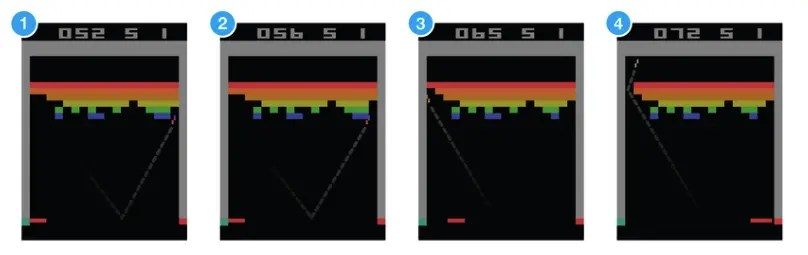Suppose you want to teach a neural network to play this game. Input to your network would be screen images, and output would be three actions: left, right or fire (to launch the ball). It would make sense to treat it as a classification problem – for each game screen you have to decide, whether you should move left, right or press fire. Sounds straightforward? Sure, but then you need training examples, and a lots of them. Of course you could go and record game sessions using expert players, but that’s not really how we learn. We don’t need somebody to tell us a million times which move to choose at each screen. We just need occasional feedback that we did the right thing and can then figure out everything else ourselves.
This is the task reinforcement learning tries to solve. Reinforcement learning lies somewhere in between supervised and unsupervised learning. Whereas in supervised learning one has a target label for each training example and in unsupervised learning one has no labels at all, in reinforcement learning one has sparse and time-delayed labels – the rewards. 基于这些收益，agent 必须学会在环境中如何行动。

# Markov Decision Process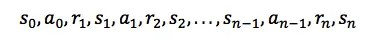# Discounted Future Reward

To perform well in the long-term, we need to take into account not only the immediate rewards, but also the future rewards we are going to get. How should we go about that?

Given one run of the Markov decision process, we can easily calculate the total reward for one episode:Given that, the total future reward from time point t onward can be expressed as: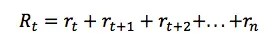But because our environment is stochastic, we can never be sure, if we will get the same rewards the next time we perform the same actions. The more into the future we go, the more it may diverge. For that reason it is common to use discounted future reward instead:Here γ is the discount factor between 0 and 1 – the more into the future the reward is, the less we take it into consideration. It is easy to see, that discounted future reward at time step t can be expressed in terms of the same thing at time step t+1:If we set the discount factor γ=0, then our strategy will be short-sighted and we rely only on the immediate rewards. If we want to balance between immediate and future rewards, we should set discount factor to something like γ=0.9. If our environment is deterministic and the same actions always result in same rewards, then we can set discount factor γ=1.

A good strategy for an agent would be to always choose an action that maximizes the (discounted) future reward.

# Q-learning

In Q-learning we define a function Q(s, a) representing the maximum discounted future reward when we perform action a in state s, and continue optimally from that point on.

**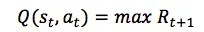**The way to think about Q(s, a) is that it is “the best possible score at the end of the game after performing action a in state s“. It is called Q-function, because it represents the “quality” of a certain action in a given state.

This may sound like quite a puzzling definition. How can we estimate the score at the end of game, if we know just the current state and action, and not the actions and rewards coming after that? We really can’t. But as a theoretical construct we can assume existence of such a function. Just close your eyes and repeat to yourself five times: “Q(s, a) exists, Q(s, a) exists, …”. Feel it?

If you’re still not convinced, then consider what the implications of having such a function would be. Suppose you are in state and pondering whether you should take action a or b. You want to select the action that results in the highest score at the end of game. Once you have the magical Q-function, the answer becomes really simple – pick the action with the highest Q-value!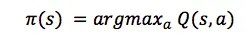Here π represents the policy, the rule how we choose an action in each state.

OK, how do we get that Q-function then? Let’s focus on just one transition <s, a, r, s’>. Just like with discounted future rewards in the previous section, we can express the Q-value of state s and action a in terms of the Q-value of the next state s’.This is called the Bellman equation. If you think about it, it is quite logical – maximum future reward for this state and action is the immediate reward plus maximum future reward for the next state.

The main idea in Q-learning is that we can iteratively approximate the Q-function using the Bellman equation. In the simplest case the Q-function is implemented as a table, with states as rows and actions as columns. The gist of the Q-learning algorithm is as simple as the following:α in the algorithm is a learning rate that controls how much of the difference between previous Q-value and newly proposed Q-value is taken into account. In particular, when α=1, then two Q[s,a] cancel and the update is exactly the same as the Bellman equation.

The maxa’ Q[s’,a’] that we use to update Q[s,a] is only an approximation and in early stages of learning it may be completely wrong. However the approximation get more and more accurate with every iteration and it has been shown, that if we perform this update enough times, then the Q-function will converge and represent the true Q-value.

# Deep Q Network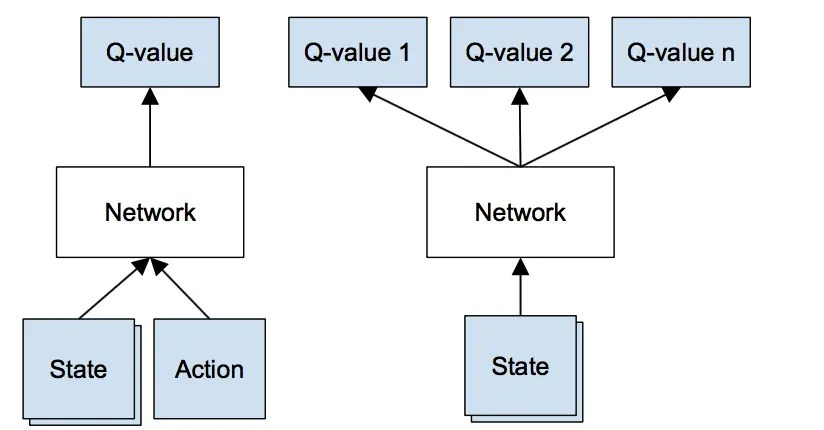DeepMind 使用的深度神经网络架构如下：1. 对当前的状态 s 执行前向传播，获得对所有行动的预测 Q-value
2. 对下一状态 s’ 执行前向传播，计算网络输出最大操作：max_{a’} Q(s’, a’)
3. 设置行动的 Q-value 目标值为 r + γ max_{a’} Q(s’, a’)。使用第二步的 max 值。对所有其他的行动，设置为和第一步返回结果相同的 Q-value 目标值，让这些输出的误差设置为 0
4. 使用反向传播算法更新权重

# Exploration-Exploitation

Q-learning 试着解决 credit assignment 问题——将受益按时间传播，直到导致获得受益的实际的关键决策点为止。但是我们并没有解决 exploration-exploitation 困境……

# Deep Q-learning 算法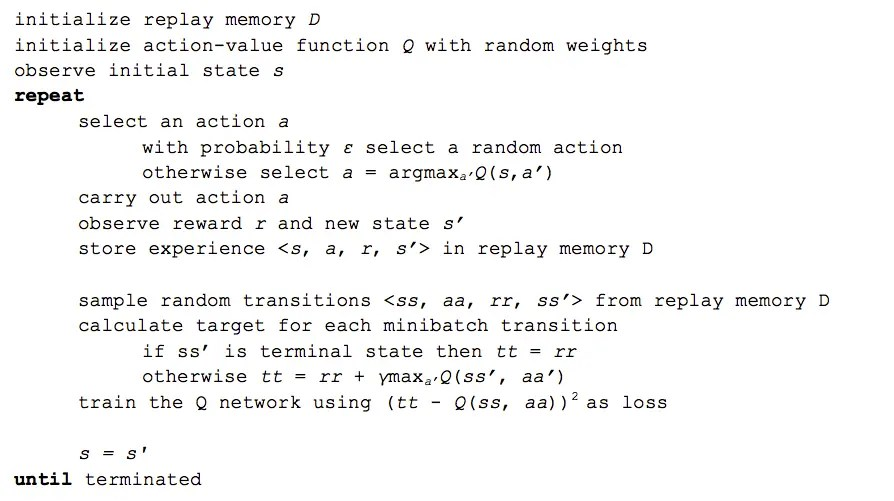DeepMind 其实还使用了很多的技巧来让系统工作得更好——如 target network、error clipping、reward clipping 等等，这里我们不做介绍。

# Final notes

Many improvements to deep Q-learning have been proposed since its first introduction – Double Q-learning, Prioritized Experience Replay, Dueling Network Architecture and extension to continuous action space to name a few. For latest advancements check out the NIPS 2015 deep reinforcement learning workshop and ICLR 2016 (search for “reinforcement” in title). But beware, that deep Q-learning has been patented by Google.

It is often said, that artificial intelligence is something we haven’t figured out yet. Once we know how it works, it doesn’t seem intelligent any more. But deep Q-networks still continue to amaze me. Watching them figure out a new game is like observing an animal in the wild – a rewarding experience by itself.

# Credits

Thanks to Ardi Tampuu, Tanel Pärnamaa, Jaan Aru, Ilya Kuzovkin, Arjun Bansal and Urs Köster for comments and suggestions on the drafts of this post.

# Excerpts from the Grothendieck-Serre CorrespondenceMatt Baker's Math Blog

Like many fellow mathematicians, I was very sad to hear the news that Alexander Grothendieckpassed away yesterday.The word “genius” is overused; or rather, does not possess sufficiently fine gradations.  I know quite a few mathematical geniuses, but Grothendieck was a singularity.  His ideas were so original, so profound, and so revolutionary – and he had so many of them! – that I will not even attempt to summarize his contributions to mathematics here.  Rather, I thought that I would share some of my favorite passages from the fascinating Grothendieck-Serre Correspondence, published in a bilingual edition by the AMS and SMF.   They illuminate in brief flashes what made Grothendieck so extraordinary — but also human.  They also illustrate how influential Serre was on Grothendieck’s mathematical development.  Before I begin, here is a quote from another wonderful book, Alexander Grothendieck: A Mathematical Portrait, edited by Leila Schneps:

…the…

View original post 2,863 more words

# 254A announcement: Analytic prime number theoryWhat's new

In the winter quarter (starting January 5) I will be teaching a graduate topics course entitled “An introduction to analytic prime number theory“. As the name suggests, this is a course covering many of the analytic number theory techniques used to study the distribution of the prime numbers $latex {{mathcal P} = {2,3,5,7,11,dots}}&fg=000000$. I will list the topics I intend to cover in this course below the fold. As with my previous courses, I will place lecture notes online on my blog in advance of the physical lectures.

The type of results about primes that one aspires to prove here is well captured by Landau’s classical list of problems:

1. Even Goldbach conjecture: every even number $latex {N}&fg=000000$ greater than two is expressible as the sum of two primes.
2. Twin prime conjecture: there are infinitely many pairs $latex {n,n+2}&fg=000000$ which are simultaneously prime.
3. Legendre’s conjecture:…

View original post 3,947 more words

# MA 1505 Tutorial 1: Derivative

Definition of Derivative:$f^{'}(x)=\lim_{\Delta x\rightarrow 0} \frac{f(x+\Delta x)-f(x)}{\Delta x}$

Rule: Assume f(x) and g(x) are two differentiable functions, the basic rules of derivative are$(f\pm g)^{'}(x)=f^{'}(x)\pm g^{'}(x)$$(f\cdot g)^{'}(x)= f^{'}(x) g(x) + f(x)g^{'}(x)$$(f/g)^{'}(x)=(f^{'}(x)g(x)-f(x)g^{'}(x))/(g(x))^{2}$$(f\circ g)^{'}(x)=f^{'}(g(x))g^{'}(x)$

Definition of Critical Point:$x_{0}$ is called a critical point of f(x), if$f^{'}(x_{0})=0.$

If$f^{'}(x)>0$ on some interval I, then f(x) is increasing on the interval I. Similarly, if$f^{'}(x)<0$ on some interval I, then f(x) is decreasing on the interval I.

Tangent Line: Assume f(x) is a differentiable function on the interval I, then the tangent line of f(x) at the point$x_{0}\in I$ is$y-f(x_{0})=f^{'}(x_{0})(x-x_{0}),$ where$f^{'}(x_{0})$ is the slope of the tangent line.

Derivative of Parameter Functions: Assume y=y(t) and x=x(t), the derivative$y^{'}(x)$ is$y^{'}(t)/x^{'}(t),$ because the Chain Rule of derivatives.

Question 1. Calculate the tangent line of the curve$x^{\frac{1}{4}} + y^{\frac{1}{4}}=4$ at the point (16,16).

Method (i). Take the derivative of the equation$x^{\frac{1}{4}}+y^{\frac{1}{4}}=4$ at the both sides, we get$\frac{1}{4}x^{-\frac{3}{4}} + \frac{1}{4}y^{-\frac{3}{4}} y^{'}=0.$

Assume x=y=16, we have the derivative$y^{'}(16)=-1.$ That means the tangent line of the curve at the point (16,16) is y-16=-(x-16). i.e. y=-x+32.

Method (ii). From the equation, we know$y(x)=(4-x^{\frac{1}{4}})^{4}$, then calculating the derivative directly. i.e.$y^{'}(x)=4(4-x^{\frac{1}{4}})^{3}\cdot (-1)\cdot \frac{1}{4}x^{-\frac{3}{4}}$

Therefore,$y^{'}(16)=-1.$

Method (iii). Making the substitution$x=4^{4}\cos^{8}\theta, y=4^{4}\sin^{8}\theta,$ then (16,16) corresponds to$\theta=\pi/4.$ From the derivative of the parameter functions, we know$\frac{dy}{dx}= \frac{dy/d\theta}{dx/d\theta}=\frac{4^{4}\cdot 8\sin^{7}\theta\cdot \cos\theta}{4^{4}\cdot 8\cos^{7}\theta\cdot (-\sin\theta)}$

If we assume$\theta=\pi/4,$ then$y^{'}(16)=-1.$

Method (iv). Geometric Intuition. Since the equation$x^{\frac{1}{4}}+y^{\frac{1}{4}}=4$ is a symmetric graph with the line y=x, and (16,16) is also on the symmetric line. Therefore, the slope of the curve at the point (16,16) is -1. Hence, the tangent line is y=-x+32.

Question 2. Let$y=(1+x^{2})^{-2}$ and$x=\cot \theta.$ Find dy/dx and express your answer in terms of$\theta.$

Method (i).$y=\frac{1}{1+x^{2}}= \sin^{2}\theta$$\frac{dy}{dx}=\frac{dy/d\theta}{dx/d\theta} = \frac{2\sin\theta \cos \theta}{-\sin^{-2}\theta}= - \sin^{2}\theta\sin2\theta.$

Method (ii).$\frac{dy}{dx}=-\frac{2x}{(1+x^{2})^{2}} = -\frac{2\cot \theta}{(1+\cot^{2}\theta)^{2}}=-\sin^{2}\theta\sin 2\theta.$

# Manjul Bhargava and his 290 theoremFight with Infinity

ICM 2014今天在韩国首尔召开。正如之前所预测的那样，Manjul Bhargava获得了2014年的Fields Medal. 一同获奖的还有Artur Avila, Martin HairerMaryam Mirzakhani.

1.

(1.1)Fermat集中研究了用2元2次整系数多项式表示素数$latex p$的问题，并发现

(1.2)由Fermat二平方和定理开始，Euler等数学家获得了一系列经典结果。
(Fermat二平方和定理, 由Euler证明) 若$latex f(x,y)=x^2+y^2$，则自然数$latex k in R_f$当且仅当$latex k$的奇素因子(若有)均形如$latex 4a+1$。
(Lagrange四平方和定理) 若$latex f(x,y,z,w)=x^2+y^2+z^2+w^2$，则$latex R_f=Bbb N$。
(Legendre三平方和定理) 若$latex f(x,y,z)=x^2+y^2+z^2$，则自然数$latex k in R_f$当且仅当$latex k$不能写成$latex 2^{2a}(8b+7)$的形式。
(1.3) 平方数有一类推广，即所谓的多边形数：填满正多边形内部的点的个数。
(Gauss三角数定理，“Eureka定理”)令$latex f(x,y,z)=frac{x(x+1)}{2}+frac{y(y+1)}{2}+frac{z(z+1)}{2}$，则$latex R_f=Bbb N$。

(Fermat多边形数定理，由Cauchy证明) 任意自然数均可表示为不超过$latex n$个$latex n$边形数之和。
(1.4)从Lagrange四平方和定理出发，我们也可以研究高次幂多项式的表示问题：
(Waring问题，由Hilbert解决) 给定$latex k geq 2$，$latex f=sum_{1 leq i leq g} x_i^k$。对于充分大的$latex g$，$latex R_f=Bbb N$。

View original post 1,244 more words

# Lindenstrauss, Ngo, Smirnov, VillaniWhat's new

As is now widely reported, the Fields medals for 2010 have been awarded to Elon Lindenstrauss, Ngo Bao Chau, Stas Smirnov, and Cedric Villani. Concurrently, the Nevanlinna prize (for outstanding contributions to mathematical aspects of information science) was awarded to Dan Spielman, the Gauss prize (for outstanding mathematical contributions that have found significant applications outside of mathematics) to Yves Meyer, and the Chern medal (for lifelong achievement in mathematics) to Louis Nirenberg. All of the recipients are of course exceptionally qualified and deserving for these awards; congratulations to all of them. (I should mention that I myself was only very tangentially involved in the awards selection process, and like everyone else, had to wait until the ceremony to find out the winners. I imagine that the work of the prize committees must have been extremely difficult.)

Today, I thought I would mention one…

View original post 3,867 more words

# Avila, Bhargava, Hairer, MirzakhaniWhat's new

The 2014 Fields medallists have just been announced as (in alphabetical order of surname) Artur Avila, Manjul Bhargava, Martin Hairer, and Maryam Mirzakhani (see also these nice video profiles for the winners, which is a new initiative of the IMU and the Simons foundation). This time last year, I wrote a blog post discussing one result from each of the 2010 medallists; I thought I would try to repeat the exercise here, although the work of the medallists this time around is a little bit further away from my own direct area of expertise than last time, and so my discussion will unfortunately be a bit superficial (and possibly not completely accurate) in places. As before, I am picking these results based on my own idiosyncratic tastes, and are not necessarily the “best” work of these medallists.

Artur Avila works in dynamical systems and in the…

View original post 1,412 more words

# 245A: Problem solving strategiesWhat's new

This is going to be a somewhat experimental post. In class, I mentioned that when solving the type of homework problems encountered in a graduate real analysis course, there are really only about a dozen or so basic tricks and techniques that are used over and over again. But I had not thought to actually try to make these tricks explicit, so I am going to try to compile here a list of some of these techniques here. But this list is going to be far from exhaustive; perhaps if other recent students of real analysis would like to share their own methods, then I encourage you to do so in the comments (even – or especially – if the techniques are somewhat vague and general in nature).

View original post 6,008 more words

# The parity problem obstruction for the binary Goldbach problem with bounded errorWhat's new

Two of the most famous open problems in additive prime number theory are the twin prime conjecture and the binary Goldbach conjecture. They have quite similar forms:

• Twin prime conjecture The equation $latex {p_1 – p_2 = 2}&fg=000000$ has infinitely many solutions with $latex {p_1,p_2}&fg=000000$ prime.
• Binary Goldbach conjecture The equation $latex {p_1 + p_2 = N}&fg=000000$ has at least one solution with $latex {p_1,p_2}&fg=000000$ prime for any given even $latex {N geq 4}&fg=000000$.

In view of this similarity, it is not surprising that the partial progress on these two conjectures have tracked each other fairly closely; the twin prime conjecture is generally considered slightly easier than the binary Goldbach conjecture, but broadly speaking any progress made on one of the conjectures has also led to a comparable amount of progress on the other. (For instance, Chen’s theorem has a version for the twin prime conjecture, and a version…

View original post 1,123 more words

# Mac OS X下MATLAB R2012b安装及破解

http://blog.sina.com.cn/s/blog_559d66460101caur.html

## Mac OS X下MATLAB R2012b安装及破解 (Updated on 10/19/2013)(2013-03-08 05:28:16)

【转载请注明出处！！！】

1. 下载 MATLAB R2012b 安装包。安装文件的大小约为5G。之前的下载地址都失效了，为了方便大家，po主自己传了一份到百度网盘。因为度娘不允许单个文件大于4G，所以压成了5 parts，请把5 parts都下完后再解压… 侵删，勿跨省跨国追捕…

Part 1: http://pan.baidu.com/s/15lLMe    提取密码：ygxm
Part 2: http://pan.baidu.com/s/1dFl4    提取密码：naxi
Part 3: http://pan.baidu.com/s/19V4cW    提取密码：zrwu

Part 4: http://pan.baidu.com/s/1FvjiY    提取密码：ag8t
Part 5: http://pan.baidu.com/s/1899jD    提取密码：f85x
PS：大家随便下，po主不设权限不收钱，不用谢，作为交换请不要关掉页面的background music，让po主私心promote一下G-Dragon的音乐各种风格都有，歌曲排序大概是普通青年->文艺青年->黑泡青年，请根据喜好自行切换2. 下载 MATLAB R2012b 安装密钥/License。地址：http://vdisk.weibo.com/s/sSDYb （微博快被@爆了… 改天传一份到度娘去…）。解压后安置在某处待用。
3. 双击MATLAB安装文件(.iso file)，选择 Install for Mac。进入以下画面时，点选 Install without using the Internet （离线安装），然后点击Next
4. 在这一步中选择 I have the File Installation Key for my license。在前面下载并解压的安装密钥文件夹中，打开文件“MatLab R2012b 安装密钥.txt”，复制任意一个密钥，粘贴到输入框中。据说不同长度的license代表所含的组件数量不一样。点击Next
5. 在这一步中，根据自己的需要，选择安装类型。普通用户的话选Typical即可，高端用户可选择自定义安装Custom。然后Next
7. 之后就开始安装了。Enjoy the free MATLAB on your Mac!
【Update】其它问题：
(1) A server line could not be found in your license file. You will have to manually edit the SERVER line in /Applications/MATLAB_R2012b.app/licenses/network.lic
PS：这个解决方法来自。因为我之前已经装了Xcode，虽然没有特别去设置什么，但没有碰到这个问题。有这个问题的小伙伴们如果没有装Xcode，就先装Xcode，还是有问题的话就装下面这个patch。

Notes for the Mac Platform
A patch is required to add support for Xcode 4.6, 4.5, 4.4 and 4.3. See Solution 1-FR6LXJ for the patch and installation instructions.

References:
 http://blog.sina.com.cn/s/blog_c29649af0101f5g4.html
 http://www.mathworks.co.uk/support/solutions/en/data/1-FR6LXJ/

# [转]DJVU格式电子图书介绍bestorworst

———————-

djvu是一种电子文档格式，于1996年被美国AT&T实验室研制成功。与我们熟悉的pdf、ppt等一样，djvu文件中存储了文字、图片等信息，可通过软件进行此类文档的制作、分享、阅读操作。

djvu的技术特色，即为什么要选择djvu？

djvu没有ppt与pdf，甚至没有pdg更大众化，但是它的技术特点确是其先天优势，具体来讲有以下几点：

1.双层打印

2.超级强大的压缩功能

3.适合网络分享

DJVU阅读器：WinDjView 0.4.1 下载

View original post

# [转载] 尤老师的乒乓球之路

【 以下文字转载自 D_Maths 讨论区 】

【 原文由 gfzhang 所发表 】

— ※ 来源:．南京大学小百合站 http://bbs.nju.edu.cn [FROM: 202.119.34.246] —

※ 来源:．南京大学小百合站 http://bbs.nju.edu.cn [FROM: 123.114.37.153]

# LaTeX 作图工具介绍

LaTeX 作图工具介绍logiclife

LaTeX 虽然在公式编辑上远胜任于 Office，但对于作图，初学者可能会觉得门槛较高而对 LaTeX 产生畏难情绪。不过，如果掌握了恰当的工具，LaTeX 下的作图仍然很方便且优于 Office。

• 利用命令绘图

1. tikz，非常强大的作图宏包，几乎可以画任何图形。甚至可以绘制简单的函数图像。其官方使用手册的最新版厚达726页。网上也有非常多的实例展示如何用 tikz 命令绘制各种图形，例如这个网页。
2. pstricks，老牌的作图宏包，异常强大。遗憾的是不支持 pdflatex 编译，不过支持xelatex（或许反过来说更对，xelatex支持pstricks）。
3. metapost，这是在 LaTeX 诞生之初就有的绘图工具，但因为不是 LaTeX 的宏包，而只是一个外部命令行工具，使用起来不够方便。不能直接在 LaTeX 中用代码画图，而必须用 metapost 命令画好图生成 eps 或 pdf 格式的文件供 LaTeX 调用。不过， metapost 的绘图能力独步天下，大概只有 pstricks 可以与之匹敌。
4. gnuplot，外部命令行工具，绘制函数图像的不二选择。提供和 LaTeX 的接口。这里是一个很好的简明入门教程。
5. xy-pic（其实宏包名为xy），如果是画交换图，特别是范畴论中的图形，使用 xy 宏包会极为方便。但画结构性不那么好的图形则比较麻烦。
6. bussproof，写 Gentzen 式树状逻辑推演极为方便。
7. qtree，画 tableau 证明树或语法分析树极为方便，但树枝没有箭头。
8. xy-ling，另一个画树状图的宏包，极其灵活，处理语言学中各种语法分析树不在话下。

• 利用 GUI 绘图软件绘图

1. Inkscape，非常强大的矢量绘图软件，可实现很多复杂的效果，跨平台，且支持多种文件格式保存。Ubuntu 可通过源安装。没有特别声明支持 LaTeX，但实际上所绘图片可以直接存成 tex 格式（其代码利用了 pstricks 宏包），也可以存成 pdf 文件，然后在保存选项中选择包含 LaTeX 代码（用于处理图片中的公式），Inkscape 会生成一个名为<image>.pdf_tex的文件，最后在 LaTeX 主文档中使用 input 命令包含这个文件即可。详见这个文档说明。如果不需要绘制函数图形，Inkscape 是这里所列的绘图软件中绘图能力最强的。
2. Ipe，比 Inkscape 小巧，因而绘图功能也较弱，但如果只需要绘制简单图形，也够用了。不能导出为 tex 代码，直接生成 eps 或 pdf 格式图片供 LaTeX 文档调用，能自动剪裁图片大小，去掉白边。跨平台。Ubuntu 可通过源安装。Linux 下必须通过命令行启动。
3. LaTeXDraw，与 Ipe 类似。好处是在手工绘图的同时自动生成 tex 代码（利用了 pstricks 宏包）。跨平台。Ubuntu 可通过源安装。
4. XFig， 比较老牌的支持 LaTeX 的 GUI 绘图软件。手工绘图后生成 .pstex（存储图片信息）和 .pstex_t（存储图片中的公式信息）文件供 tex 主文档调用。跨平台。Ubuntu 可通过源安装。虽然不是专业的图片编辑软件，但与 Inkscapte 相比，XFig 处理简单的数学图形可能更方便。缺点是：界面丑陋，而且不支持 pdflatex 编译，要先用 latex 编译，然后转成 pdf。
5. TpX，是我接触最早的支持 LaTeX 的 GUI 绘图软件，据说是一个经济学家因为要出书，图片太多，不方便处理，所以自己动手写了这个软件。与 Ipe 类似。小巧，方便。缺点是只支持 Windows。
6. GeoGebra，专门绘制函数图像，支持导出为 tikz 或 pstricks 代码，跨平台。Ubuntu 可通过源安装。
7. Dia，专门绘制流程图，支持导出为…

View original post 10 more words